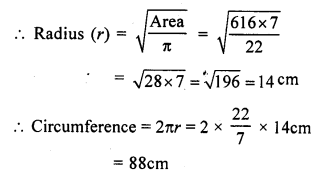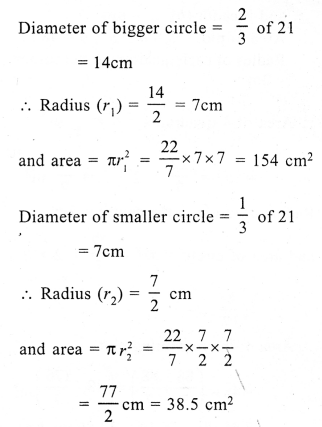# RS Aggarwal Class 7 Solutions Chapter 20 Mensuration Ex 20F

In this chapter, we provide RS Aggarwal Solutions for Class 7 Chapter 20 Mensuration Ex 20F for English medium students, Which will very helpful for every student in their exams. Students can download the latest RS Aggarwal Solutions for Class 7 Chapter 20 Mensuration Ex 20F Maths pdf, free RS Aggarwal Solutions Class 7 Chapter 20 Mensuration Ex 20F Maths book pdf download. Now you will get step by step solution to each question.

### RS Aggarwal Solutions for Class 7 Chapter 20 Mensuration Ex 20F Download PDF

Question 1.
Solution:
(i) Radius of the circle (r) = 21 cmQuestion 2.
Solution:
(i) Diameter of the circle = 28 cmQuestion 3.
Solution:
Circumference of a circle = 264 cmQuestion 4.
Solution:
Circumference of the circle (c) = 35.2 mQuestion 5.
Solution:
Area of the circle = 616 cm²Question 6.
Solution:
Area of a circle = 1386 m²Question 7.
Solution:
Ratio in the radii of two circles = 4 : 5
Let radius of first circle (r1) = 4x
and radius of the second circle (r2) = 5xQuestion 8.
Solution:
Length of rope (r) = 21 m
Area of the circle = πr² = 227 x 21 x 21 m² = 1386 m²
The horse will graze on 1386 m² area

Question 9.
Solution:
Area of a square made of a wire = 121 cm²
Side = √Area = √121 = 11 m
Perimeter of wire = 4 x side = 4 x 11 = 44 cm
Circumference of circular wire = 44 cmQuestion 10.
Solution:
Radius of circular wire = 28 cm
Circumference = 2πr = 2 x 227 x 28 cm = 176 cm
Perimeter of the square formed by this wire = 176 cm
Side (a) = 1764 = 44 cm .
Area of square so formed = a² = (44)² cm² = 1936 cm²

Question 11.
Solution:
Length of rectangular sheet (l) = 34 cm
and breadth (b) = 24 cm
Area = l x b = 34 x 24 cm² = 816 cm²
Diameter of one button = 3.5 cm
and area of one button = πr²Area of 64 buttons = 9.625 x 64 cm² =616 cm²
Area of remaining sheet = 816 – 616 = 200 cm²

Question 12.
Solution:
Length of ground (l) = 90 m
and breadth (b) = 32 m
Area = l x b = 90 x 32 m² = 2880 m²
Radius of circular tank (r) = 14 m= 616 m²
Area of remaining portion = 2880 – 616 = 2264 m²
Rate of turfing = Rs. 50 per sq.m.
Total cost = Rs. 50 x 2264 = Rs. 113200

Question 13.
Solution:
Each side of square = 14 cm
Area of square = a² = 14 x 14 = 196 cm²
Radius of each circle at each corner of square = 142 = 7 cmArea of shaded portion = Area of square – area of 4 quadrants
= 196 – 154 = 42 cm²

Question 14.
Solution:
Length of field = 60 m
Length of rope = 14 m
Area covered by the horseQuestion 15.
Solution:
Diameter of largest circle (outer circle) = 21 cmArea of shaded portion = 346.5 – (154 + 38.5) = (346.5 – 192.5) = 154 cm²

Question 16.
Solution:
Length of plot (l) = 8m
Area of plot = l x b = 8 x 6 = 48 m²All Chapter RS Aggarwal Solutions For Class 7 Maths

—————————————————————————–

All Subject NCERT Exemplar Problems Solutions For Class 7

All Subject NCERT Solutions For Class 7

*************************************************

I think you got complete solutions for this chapter. If You have any queries regarding this chapter, please comment on the below section our subject teacher will answer you. We tried our best to give complete solutions so you got good marks in your exam.

If these solutions have helped you, you can also share rsaggarwalsolutions.in to your friends.